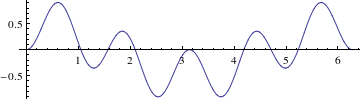## Section10.3Inner Products of Harmonic Functions

What happens if you multiply two different trig functions, for example $$\sin(2\theta)\sin(3\theta)\text{,}$$ as shown in Figure 10.3.1. It looks about as you might expect, with the overall structure (often called an envelope) of a large wave controlled by the function with the longer wavelength $$\sin(2\theta)$$ and with smaller “wiggles” controlled by the function with the shorter wavelength $$\sin(3\theta)\text{.}$$Figure 10.3.1. The graph of $$y=\sin(2\theta)\sin(3\theta)\text{.}$$

For Fourier series, what we will care about is the area under this graph. What is the integral of this combined function? Hard to tell, but there's about as much area above the axis as below, so zero would be plausible guess, which turns out to be correct. This central identity underlies all of Fourier theory.

It is in fact not that difficult to evaluate such integrals in closed form. Using technology or integral tables, we find the indefinite integral

\begin{align} \int \cos(m\theta) \cos(n\theta) \,d\theta \amp = \frac{m\,\sin(m\theta)\cos(n\theta)-n\,\cos(m\theta)\sin(n\theta)} {m^2-n^2}\tag{10.3.1} \end{align}

for $$m\ne n\text{.}$$ There are similar formulas for the various combinations of sines and cosines. You should notice that we will need to be careful when $$m\ne n\text{.}$$

For Fourier series, we care about the cases where $$m$$ and $$n$$ are both integers, and we want the definite integral over an entire period; with these assumptions, we find

\begin{align} \int_0^{2\pi} \sin(m\theta) \sin(n\theta) \,d\theta \amp = 0 ,\tag{10.3.2}\\ \int_0^{2\pi} \sin(m\theta) \cos(n\theta) \,d\theta \amp = 0 ,\tag{10.3.3}\\ \int_0^{2\pi} \cos(m\theta) \cos(n\theta) \,d\theta \amp = 0\text{.}\tag{10.3.4} \end{align}

Of course, if $$m=n\ne0$$ is an integer, we get different results, namely

\begin{align} \int_0^{2\pi} \sin^2(m\theta) \,d\theta \amp = \pi ,\tag{10.3.5}\\ \int_0^{2\pi} \sin(m\theta) \cos(m\theta) \,d\theta \amp = 0 ,\tag{10.3.6}\\ \int_0^{2\pi} \cos^2(m\theta) \,d\theta \amp = \pi\text{.}\tag{10.3.7} \end{align}

To complete this list, we consider the case $$m=0\text{.}$$ Since $$\sin(0\,\theta)$$ is the zero function, it is enough to examine $$\cos(0\,\theta)=1\text{.}$$ We get one last formula:

\begin{equation} \int_0^{2\pi} \cos^2(0\,\theta) \,d\theta = 2\pi\text{.}\tag{10.3.8} \end{equation}

### Activity10.3.1.Integrals of products of harmonic functions.

Use Euler's formula (2.5.1) to find the values of the definite integrals in this section by hand.

### Activity10.3.2.Changing the period of integrals of harmonic functions.

In the definite integrals above, the variable $$x$$ is dimensionless. In practical physics problems, you often want to work with a function which is periodic on the range $$0\le y\le L\text{,}$$ where $$y$$ is a variable with dimensions of length. Use a simple change of variables to find equations analogous to the ones above.

FIXME Move content about inner products from Section 10.4 to this section.\include{complete}